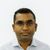# App Development

Announcements
cancel
Showing results for
Did you mean:Contributor III

## how to count data

Hi,

I am creating the sample data table  here :

 Code Category Key 112 A 001236 112 A 002589 112 A 002574 456 B 003698 456 B 007896 890 A 002365 890 A 005987 890 A 002365 342 B 001478

.i have 3 fields i want count of "key" of all code where "category" = A. I tried aggr function in expression but did not to get the expected answer.

my input is Code. for example: when I select code (112 ) it belongs to category A . so my expected answer is count of all key which has category A.

Expected result:      A =  count key = " 6 "

i have tried this expression but it gives me different answer.

Sum(Aggr(Count(Key),category)

Thanks!

Labels (1)
• ### General Question

1 Solution

Accepted SolutionsMVP

Can u try this?

``=Count({1<Category=p()>} Key)``
7 RepliesMVP

Try this,``=Count({1<Category=p()>} TOTAL Key)``Contributor III
Author

Thanks Saravanan , yes your output is correct but it didn't work for me it gave me total count key of all categories. I really appreciated.MVP

Can u try this?

``=Count({1<Category=p()>} Key)``Contributor III
Author

no, it does not work either. Thanks!MVP

Can you describe how you tried to implement the proposed solution and how your results are different from your expectation?Contributor III
Author

it worked perfectly with this sample inline table :

=Count({1<Category=p()>} Key)

Test:
Code,Category,Key
1122, A, 6
1122, A,8
1122, A, 5
456, B, 7
456, B, 1
890, A, 2
890, A, 3
789, B, 4
789, B, 9
678, C, 10
678, C, 12
]
;

Result:

A= 5

B= 4

C=2

i have huge data of 10 categories and hundreds of code , the above expression should work for  it too but dont know why it does not work.Contributor III
Author

Thanks Saravanan , it works actually i didnt put filters in set analysis. now values are correct. I really appreciated, Thanks again one more time.Tags